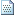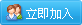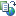PHP教程

# ASP随机数

<%=RND()%>

0.7055643

<%=INT((upperbound+1)*RND)%>

<%=INT(5+1)*RND)%>

<%=INT((upperbound – lowerbound + 1)*RND+lowerbound)%>

<%=INT((75-50+1)*RND+50)%>

<%

Pick_Greeting=INT((2+1)*RND)

Select CASE Pick_Greeting

CASE 0

Greeting=”Welcome!”

CASE 1

Greeting=”Hello!”

CASE 2

Greeting=”Happy to see you!”

END Select

%>

<%=Greeting%>

<%

RANDOMIZE

Pick_Greeting=INT((2+1)*RND)

Select CASE Pick_Greeting

CASE 0

Greeting=”Welcome!”

CASE 1

Greeting=”Hello!”

CASE 2

Greeting=”Happy to see you!”

END Select

%>

<%=Greeting%>

<%

CONST upperbound=9,iterations=100

REDIM DIST(upperbound)

RANDOMIZE

FOR i=1 to iterations

rnd_num=INT（(upperbound+1)*RND)

DIST(rnd_num)=DIST(rnd_num)&”#”

NEXT

FOR i=0 to upperbound

%>

<%=i&” : “&DIST(i)%><BR>

<%

NEXT

%>文章来自: 本站原创Tags: